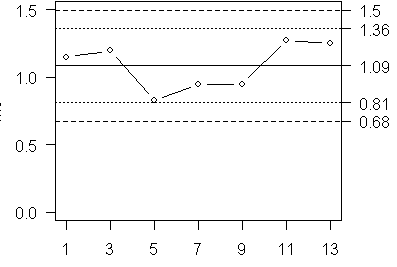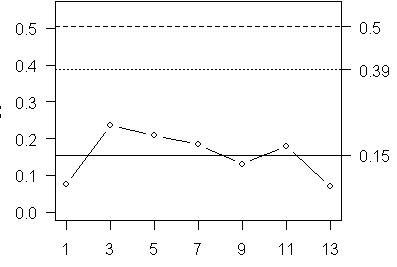## 2007-03-02

On the web page

you were asked to calculate an XBAR-S control chart. Here is the data for that problem.

Week01 1.097 1.204
Week03 1.030 1.362
Week05 0.682 0.978
Week07 0.820 1.080
Week09 1.042 0.858
Week11 1.398 1.146
Week13 1.301 1.204


Here are the summary statistics.

             Mean Stdev
Week01 1.151 0.076
Week03 1.196 0.235
Week05 0.830 0.209
Week07 0.950 0.184
Week09 0.950 0.130
Week11 1.272 0.178
Week13 1.252 0.069


The mean of the seven means is 1.09 and the mean of the seven standard deviations is 0.15. For subgroups of size 2, the constants are

A3=2.659,    B3=0,    B4=3.267.


The upper and lower control limits for the XBAR chart are

1.09+2.659*0.15=1.49 and
1.09-2.659*0.15=0.69.


The warning limits are

1.09+2.659*0.15*2/3=1.36 and
1.09-2.659*0.15*2/3=0.82.


Here is the control chart for the means (note that the numbers are slightly different because of rounding).The upper control limit for the S chart is

3.267*0.15=0.49.


The distance from the center line to the upper control limit is

0.49-0.15=0.34.


The warning limit is placed at 2/3 of the distance from the center line or

0.15+0.34*2/3=0.38.
`

Here is the S chart (note again some slight discrepancies due to rounding).You can find an earlier version of this page on my old website.